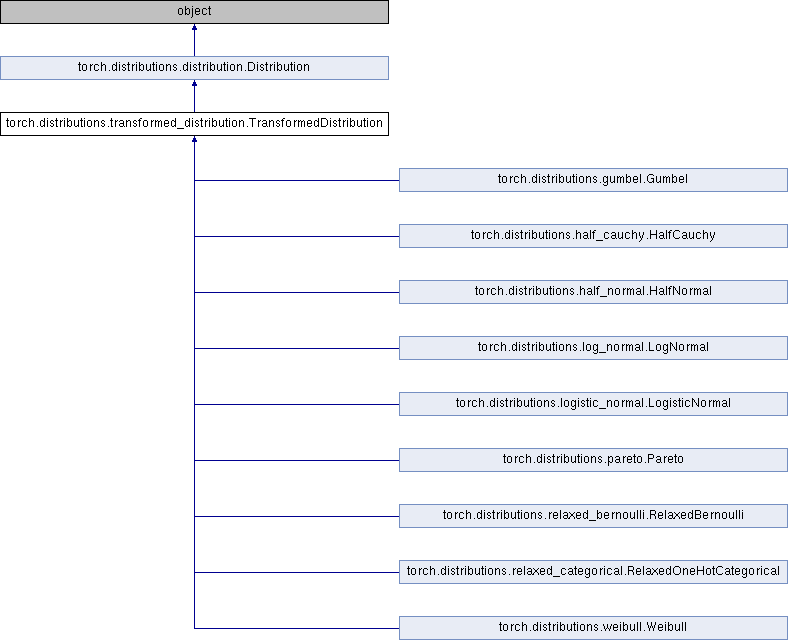Caffe2 - Python API A deep learning, cross platform ML framework
torch.distributions.transformed_distribution.TransformedDistribution Class Reference
Inheritance diagram for torch.distributions.transformed_distribution.TransformedDistribution:## Public Member Functions

def __init__ (self, base_distribution, transforms, validate_args=None)

def expand (self, batch_shape, _instance=None)

def support (self)

def has_rsample (self)

def sample (self, sample_shape=torch.Size())

def rsample (self, sample_shape=torch.Size())

def log_prob (self, value)

def cdf (self, value)

def icdf (self, value)Public Member Functions inherited from torch.distributions.distribution.Distribution
def __init__ (self, batch_shape=torch.Size(), event_shape=torch.Size(), validate_args=None)

def expand (self, batch_shape, _instance=None)

def batch_shape (self)

def event_shape (self)

def arg_constraints (self)

def support (self)

def mean (self)

def variance (self)

def stddev (self)

def sample (self, sample_shape=torch.Size())

def rsample (self, sample_shape=torch.Size())

def sample_n (self, n)

def log_prob (self, value)

def cdf (self, value)

def icdf (self, value)

def enumerate_support (self, expand=True)

def entropy (self)

def perplexity (self)

def __repr__ (self)

base_dist

transforms

## Static Public Attributes

arg_constraintsStatic Public Attributes inherited from torch.distributions.distribution.Distribution
has_rsample

has_enumerate_support

support

arg_constraintsStatic Public Member Functions inherited from torch.distributions.distribution.Distribution
def set_default_validate_args (value)

## Detailed Description

Definition at line 8 of file transformed_distribution.py.

## Member Function Documentation

 def torch.distributions.transformed_distribution.TransformedDistribution.cdf ( self, value )
```Computes the cumulative distribution function by inverting the
transform(s) and computing the score of the base distribution.
```

Definition at line 132 of file transformed_distribution.py.

 def torch.distributions.transformed_distribution.TransformedDistribution.icdf ( self, value )
```Computes the inverse cumulative distribution function using
transform(s) and computing the score of the base distribution.
```

Definition at line 145 of file transformed_distribution.py.

 def torch.distributions.transformed_distribution.TransformedDistribution.log_prob ( self, value )
```Scores the sample by inverting the transform(s) and computing the score
using the score of the base distribution and the log abs det jacobian.
```

Definition at line 102 of file transformed_distribution.py.

 def torch.distributions.transformed_distribution.TransformedDistribution.rsample ( self, sample_shape = `torch.Size()` )
```Generates a sample_shape shaped reparameterized sample or sample_shape
shaped batch of reparameterized samples if the distribution parameters
are batched. Samples first from base distribution and applies
`transform()` for every transform in the list.
```

Definition at line 90 of file transformed_distribution.py.

 def torch.distributions.transformed_distribution.TransformedDistribution.sample ( self, sample_shape = `torch.Size()` )
```Generates a sample_shape shaped sample or sample_shape shaped batch of
samples if the distribution parameters are batched. Samples first from
base distribution and applies `transform()` for every transform in the
list.
```

Definition at line 77 of file transformed_distribution.py.

The documentation for this class was generated from the following file: